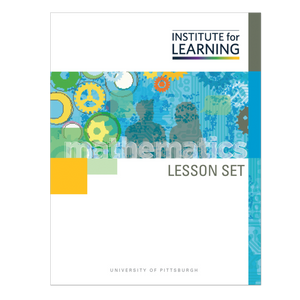# Addition Situations: Solving for the Sum (Kindergarten)

Regular price \$81.00 \$0.00 Unit price per

This lesson set provides an in-depth study of part-part-whole relationships as represented in “put together” situations and in addition equations.

Through engaging in the lessons in this lesson set, students will:

• make sense of, represent, and solve “put together” addition situations;
• identify the parts and the whole in part-part-whole relationships represented by word problems and equations;
• relate counting to addition through two strategies: counting all and counting on; and
• recognize when one strategy is more appropriate than another.

Standards: K.OA.A.1, K.OA.A.2